##### Calculus: 1001 Practice Problems For Dummies (+ Free Online Practice)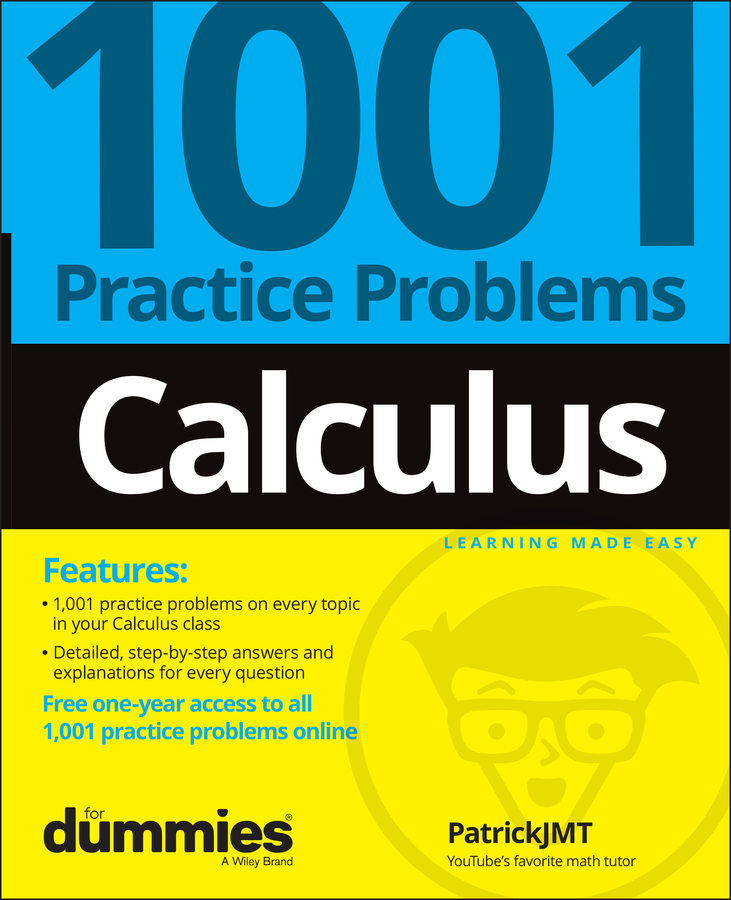At some point in your calculus life, you'll be asked to find a limit at infinity. Don't panic. Even when a limit expression looks tricky, you can use a number of techniques to change it so that you can plug in and solve it.

The following practice problems require you to use some of these techniques, including conjugate multiplication, FOILing, finding the least common denominator, simplifying, and canceling.

## Practice questions

1. Evaluate the following limit expression: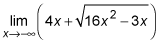2. Evaluate the following limit expression: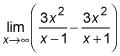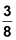So, how do you get this answer? First, put the entire expression over 1 so you can use the conjugate trick: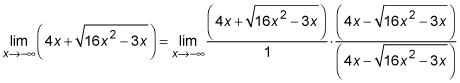Then FOIL the numerator: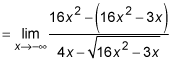Now simplify the numerator and factor out 16x2 inside the radicand: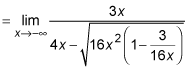Pull the 16x2 out of the square root; it becomes –4x.

You have to pull a positive out of the radicand (as always), so you pull out negative 4x because when x is negative (which it is as it approaches negative infinity), –4x is positive. Got it?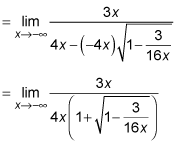Now you can cancel and plug in: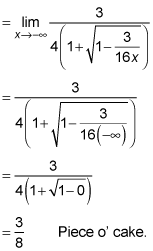To find the answer, you start by subtracting the fractions using the LCD of (x – 1)(x + 1) = x2 – 1. So: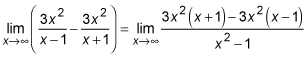Now you simplify: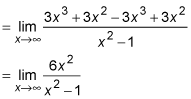Your answer is the quotient of the coefficients of x2 in the numerator and the denominator.

Here's how that works: If the degrees of the two polynomials are equal, there's a horizontal asymptote at the number you get when you divide the coefficient of the highest power term in the numerator by the coefficient of the highest power term in the denominator. This number is the answer to the limit as x approaches infinity or negative infinity.

In this case, the coefficients of x2 are 6 in the numerator and 1 in the denominator. So the quotient of the coefficients is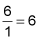Note that had you plugged in infinity in the original problem, you would have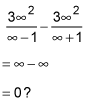It may seem strange, but infinity minus infinity does not equal 0.